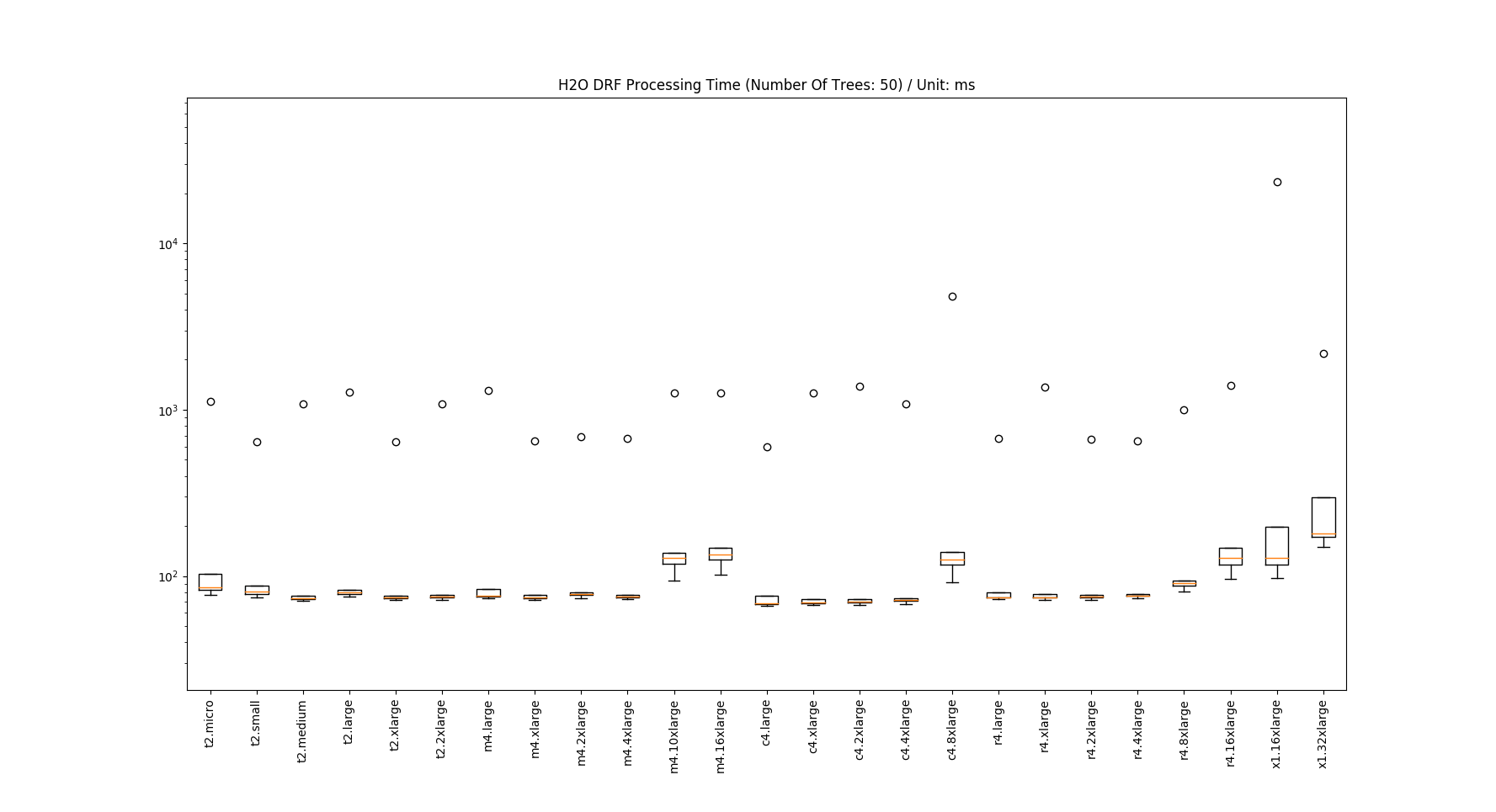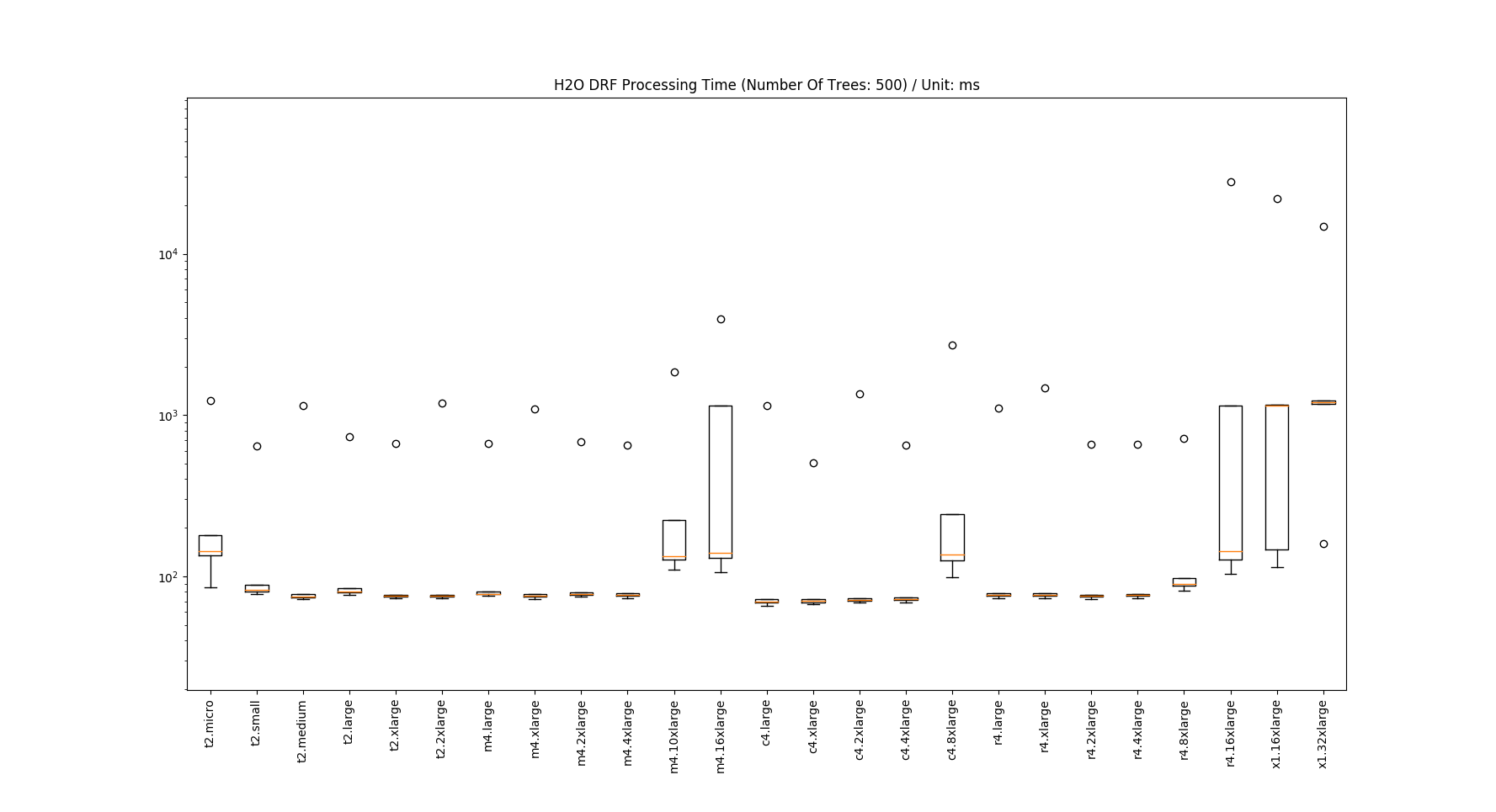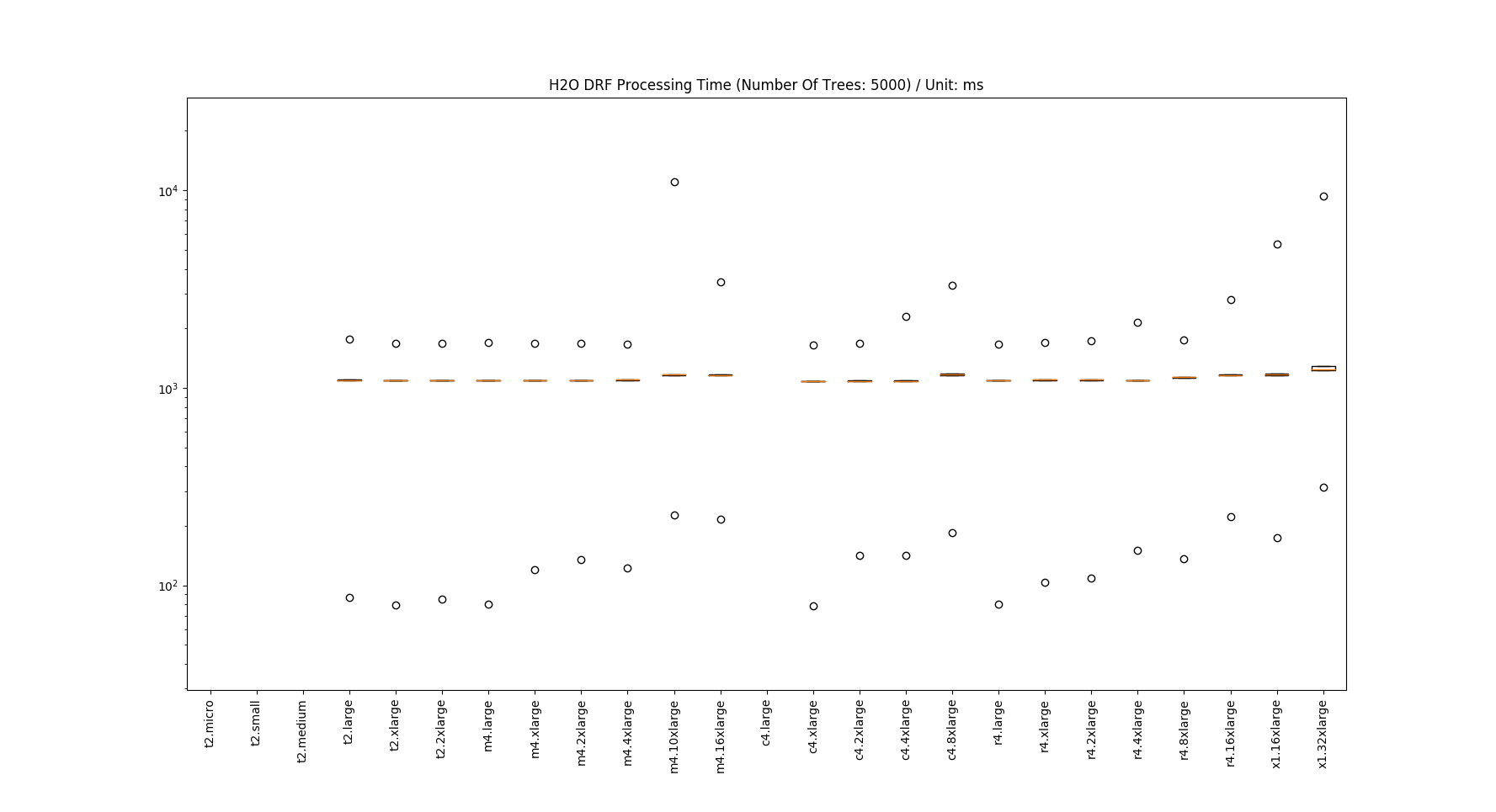# Measuring R H2O's DRF models' processing time

To measure is to know

Lord Kevin (1824 – 1903)

As we’ve been talking to many companies, we’ve found that one of common reasons that companies do not use machine learning is their fear that a machine learning model would slow their real-time transaction processing. Moreover, some companies that currently use machine learning models in their product environments wanted to know whether changing servers’ hardware specification would improve or influence their current processing time. Here, we provide processing time statistics of R H2O package’s Distributed Random Forest (DRF) models in all 25 related AWS instance types. Would running machine learning models in production take too long to process transactions real-time? Would upgrading or lowering your hardware specification improve or degrade running machine learning models in production? Let us find answers here.

# Experimentation Setup

We created 3 DRF models with different number of trees (50, 500, and 5000; max_depth is fixed at 20 and other parameters used default values) using the script here, which is almost a direct copy of H2O’s tutorial.

Then using this and this script (the first one is running the models in one instance and the second one is to automatically launch a new instance, run the first script in the instance, measure processing time periods and terminate the instance), we ran the models in AWS’ all related 25 instance types against 500 data points. While running, we measure processing time for all runs and later calculated quantile statistics to create box plots, which are presented in the next section.

# Results

## Number of trees = 50## Number of trees = 500## Number of trees = 5,000• Note that t2.micro, t2.small and t2.medium instance types could not load the model due to not enough memory.

# Analysis

We summarize important observations as follows

• Running DRF models with 50 and 500 trees for one data point typically takes about 100ms. However, running DRF models with 5,000 trees 1,000ms (1 second) with many outliers that take significantly longer time. This means that if you plan to run a DRF model in a production environment, you would want to limit the number of trees within hundreds
• Instance types with low memory might not be able to load your models as we have observed in the case where the number of trees was 5,000
• Too little memory (e.g. 1GB of t2.micro) might not be sufficient and could degrade your model's processing performance as we have observed in the case when the number of trees were 50 and 500, and the instance type was t2.miro
• Once models could be loaded, there was not much of processing performance difference across different instance types except the t2.micro case above
• Instance types with too high vCPUs and too large memory size showed degraded performance. As of now, we do not know theoretical reasons behind (perhaps the way their hardware is configured is more suitable to handle multiple tasks) but at least we do find here is that once a model could be loaded to memory, vCPUs and memory sizes do not influence processing time too much (though having a vCPU is likely to help handling multiple transactions).

To even further summarize our findings, we can say:

• Running a R H2O’s DRF model in production would take about 100ms
• Limit the number of trees within hundreds
• If you use a DRF model with tens of trees, 2 GB of memory (which is the memory size of t2.small) would be sufficient to load your model into memory and run without much performance degradation
• If hundreds of trees, we'd recommend t2.medium with 4GB of RAM and one extra vCPU
• Once a model could be loaded to memory, vCPU and memory size won’t influence too much when handling a single transaction at a time.

I hope that the findings from our experiment would help you in designing and creating a real-time transaction processing environment that uses machine learning models. Later, we will share more experiment results with different settings like multi-processing and Python machine learning models.